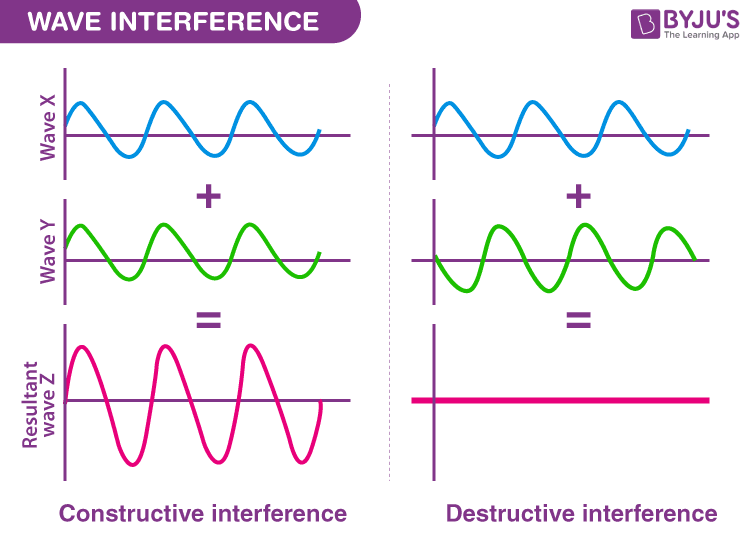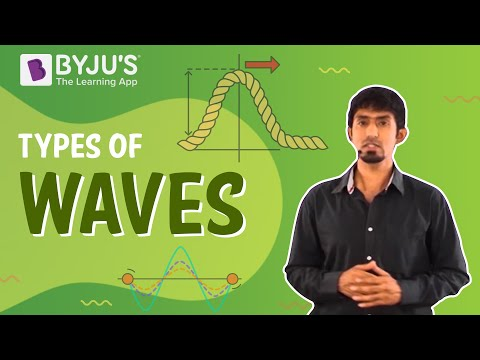# Travelling Wave

When something about the physical world changes, the information about that disturbance gradually moves outwards, away from the source, in every direction. As the information travels, it travels in the form of a wave. Sound to our ears, light to our eyes, and electromagnetic radiation to our mobile phones are all transported in the form of waves. A good visual example of the propagation of waves is the waves created on the surface of the water when a stone is dropped into a lake. In this article, we will be learning more about travelling waves.

## Describing a Wave

A wave can be described as a disturbance in a medium that travels transferring momentum and energy without any net motion of the medium. A wave in which the positions of maximum and minimum amplitude travel through the medium is known as a travelling wave. To better understand a wave, let us think of the disturbance caused when we jump on a trampoline. When we jump on a trampoline, the downward push that we create at a point on the trampoline slightly moves the material next to it downward too.

When the created disturbance travels outward, the point at which our feet first hit the trampoline recovers moving outward because of the tension force in the trampoline and that moves the surrounding nearby materials outward too. This up and down motion gradually ripples out as it covers more area of the trampoline. And, this disturbance takes the shape of a wave.

Following are a few important points to remember about the wave:

• The high points in the wave are known as crests and the low points in the wave are known as troughs.
• The maximum distance of the disturbance of the wave from the mid-point to either the top of the crest or to the bottom of a trough is known as amplitude.
• The distance between two adjacent crests or two adjacent troughs is known as a wavelength and is denoted by 𝛌.
• The time interval of one complete vibration is known as a time period.
• The number of vibrations the wave undergoes in one second is known as a frequency.
• The relationship between the time period and frequency is given as follows:
$$\begin{array}{l}T=\frac{1}{f}\end{array}$$
• The speed of a wave is given by the equation
$$\begin{array}{l}V=\lambda f\end{array}$$

### Different Types of Waves

Different types of waves exhibit distinct characteristics. These characteristics help us distinguish between wave types. The orientation of particle motion relative to the direction of wave propagation is one way the traveling waves are distinguished. Following are the different types of waves categorized based on the particle motion:

• Pulse Waves – A pulse wave is a wave comprising only one disturbance or only one crest that travels through the transmission medium.
• Continuous Waves – A continuous-wave is a waveform of constant amplitude and frequency.
• Transverse Waves – In a transverse wave, the motion of the particle is perpendicular to the direction of propagation of the wave.
• Longitudinal Waves – Longitudinal waves are the waves in which the motion of the particle is in the same direction as the propagation of the wave.

Although they are different, there is one property common between them and that is the transportation of energy. An object in simple harmonic motion has an energy of

$$\begin{array}{l}E=\frac{1}{2}kA^2\end{array}$$
. From this equation, we can concur that the energy of a wave is directly proportional to the square of the amplitude, i.e.
$$\begin{array}{l}E\propto A^2\end{array}$$
. This relationship between the energy and the amplitude is important in figuring out the damage that can be caused by the shock waves from an earthquake. As the waves spread out, they get weaker because they are distributed over more area.

### Constructive and Destructive Interference

A phenomenon in which two waves superimpose to form a resultant wave of lower, greater, or the same amplitude is known as interference. Constructive and destructive interference occurs due to the interaction of waves that are correlated with each other either because of the same frequency or because they come from the same source. The interference effects can be observed in all types of waves such as gravity waves and light waves.According to the principle of superposition of the waves, when two or more propagating waves of the same type are incidents on the same point, the resultant amplitude is equal to the vector sum of the amplitudes of the individual waves. When a crest of a wave meets a crest of another wave of the same frequency at the same point, then the resultant amplitude is the sum of the individual amplitudes. This type of interference is known as constructive interference. If a crest of a wave meets a trough of another wave, then the resulting amplitude is equal to the difference in the individual amplitudes and this is known as destructive interference.

Stay tuned to BYJU’S to learn more physics concepts with the help of interactive videos.

## Watch the video and understand longitudinal and transverse waves in detail.## Frequently Asked Questions – FAQs

### What is a pulse wave?

A pulse wave is a wave comprising only one disturbance or only one crest that travels through the transmission medium.

### What are longitudinal waves?

Longitudinal waves are the waves in which the motion of the particle is in the same direction as the propagation of the wave.

### What is superposition of waves?

According to the principle of superposition. The resultant displacement of a number of waves in a medium at a particular point is the vector sum of the individual displacements produced by each of the waves at that point.

### What is electromagnetic radiation?

Electromagnetic radiation can be defined as a form of energy that is produced by the movement of electrically charged particles travelling through a matter or vacuum or by oscillating magnetic and electric disturbance.

### What is constructive interference?

If we add two waves together by superposition the new wave has a larger amplitude but looks similar to the original waves. This situation, where the resultant wave is bigger than either of the two original waves is called constructive interference.
Test your Knowledge on Travelling Waves